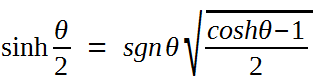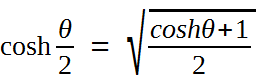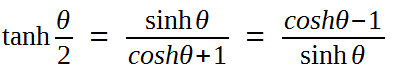# Half-Angle Formulas

Half-angle formulas can express trigonometric functions of an angle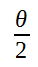in terms of functions of an angle θ.

### Half-angle formulas list: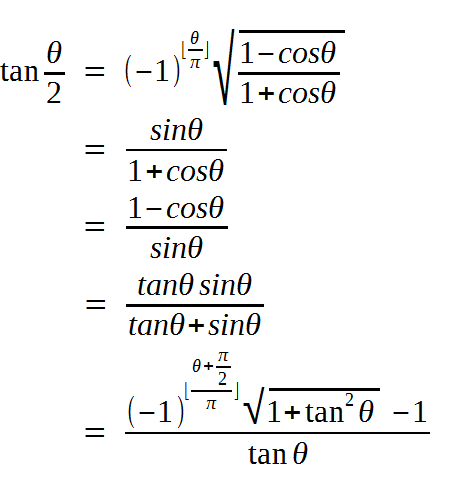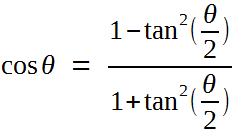### Corresponding hyperbolic function half-angle formulas list: